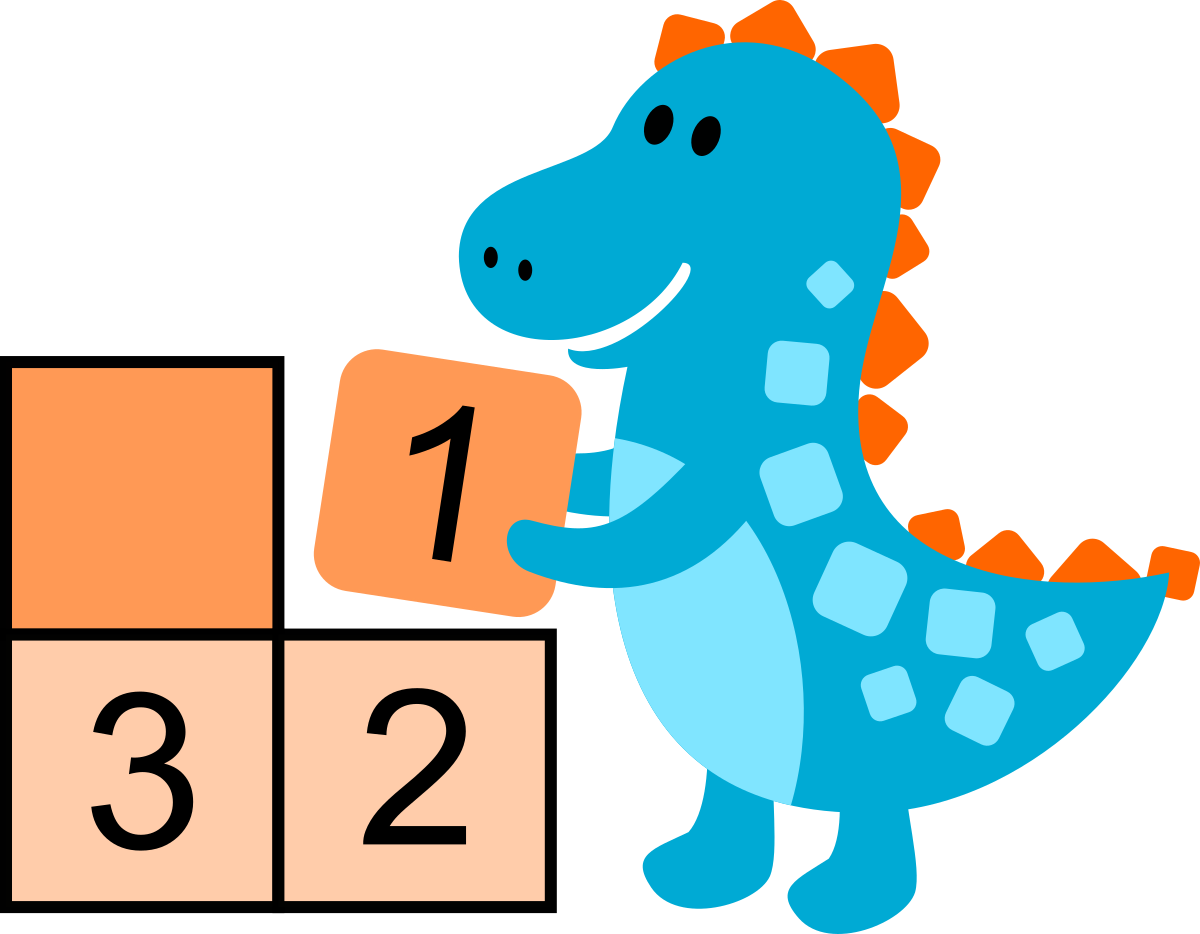Free logic and number games to exercize your brain!

## How to solve Digiblocus games

Solving techniques and strategies to find the solution of Digiblocus games.

Here are some techniques to help you find which cell contains each digit to complete the grid.

The goal is to make logical deductions and to avoid guesswork. Taking chances by putting one digit randomly can (and often will) backfire and require undoing many steps. And even if it works, it won't be as satisfying as completing the grid in a smart and logical way!### Step by step guide to solve a Digiblocus game grid

As an example, we are going to try to find the solution of an easy 5x5 grid with blocks of at most 5 digits.

Here is the playable game grid if you want to give it a try.

Note: each of the steps below demonstrates a different technique to find the only possible digit that can go into a cell. The techniques can often be combined in different ways to solve a grid. Each grid has one and only one solution, but there can be multiple ways to find that solution.

### 1. Start by filling one cell blocks.

If a block has only one cell, it must contain a 1. So we can put a 1 in the bottom right block.

Note: for each of the steps, newly found digits will be shown in GREEN.

### 2. Look for blocks with only one empty cell.

If a block has only one empty cell, then it must contain the only digit not already contained by the other cells.

Remember that blocks contain unique digits starting from 1 up to the size of the block. So a 2 cell block contains a 1 and a 2.

Note: digits that were found in previous steps will be shown in BLUE.

### 3. In each block, check if one of the missing digits can go in only cell.

The 1 in the bottom right corner touches 3 cells of the surrounding block. So the 1 in the surrounding block must be in the only other free cell.

An effective strategy is to check all missing 1s, then 2s etc. as finding a digit in a block will often help to place the same digit in other blocks.

### 4. Check if a newly found digit can help to find more digits.

The 1 that we just put in the previous step prevent 2 cells in the adjacent block from containing a 1. And the top right block also prevent another cell. So only the bottom left cell of the block can contain a 1.

### 5. Look for empty cells that are surrounded by all possible digits except one.

Below the 1 we put in the previous step, there is an empty cell that is surrounded by a 1, a 4, a 2 and a 3. The only possible digit left is thus 5.

### 6. Even empty cells can help find what digit is an adjacent cell!

The top left block has a 4 and a 5, and 3 empty cells. So the empty cells must contain a 1, a 2 and a 3.}

Even though we do not know which exact cell contains each of the 1, 2 and 3, they all touch the same cell of the adjacent block.

So we know that cell cannot contain a 1, a 2 or a 3. And since that block already contains a 4, the cell must contain a 5.

### 7. Play the "What if...?" game.

There are many times where we are presented by a range of possibilities: we know one cell must contain a 3 or a 4. Or one digit can only be in 2 or 3 cells.

To know which choice is the right one, we can test them, by asking ourselves "What if I put this digit in this cell? Would it work?".

The goal is to see if putting this digit in this cell would force us to then put other digits that would lead us into an impossible situation. If we do get to an impossible situation, then we know it wasn't the right choice, and we can eliminate it from the possibilities.

As an example, if we want to place the digit 2 in the block next to the bottom right corner, we have 3 choices: the 3 empty cells. If we put the 2 in one of the upper free cells (in RED), then that means the 2 in the block above cannot be in its lower free cell. But it cannot be in the upper free cell either because of the 2 in the top right corner. We are in an impossible situation, so our initial choice of putting the 2 in one of the upper free cells is invalid. As a result, the 2 must be in the lower free cell.

Ideally, you need to do this thinking in your head, so you don't have to actually put digits to try them, which would force you to undo them after.

And sometimes it may take quite a lot of steps before you reach an impossible situation!

### 8. Repeat until all cells are filled.

Each cell you fill give you more clues to find the digits of the other cells.

I hope these techniques will help you to solve Digiblocus games and that you will have a lot of pleasure playing them!